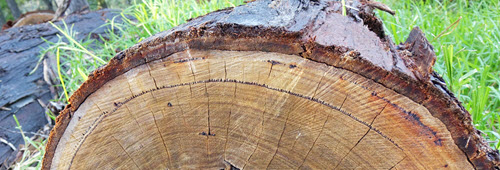# Solids of Revolution by ShellsWe can have a function, like this one:And revolve it around the y-axis to get a solid like this:Now, to find its volume we can add up "shells":Each shell has the curved surface area of a cylinder whose area is 2πr times its height:And the volume is found by summing all those shells using Integration:

Volume =
b
a

That is our formula for Solids of Revolution by Shells

These are the steps:

• sketch the volume and how a typical shell fits inside it
• integrate 2π times the shell's radius times the shell's height,
• put in the values for b and a, subtract, and you are done.

As in this example:

### Example: A Cone!

Take the simple function y = b − x between x=0 and x=bRotate it around the y-axis ... and we have a cone!Now let us imagine a shell inside:What is the shell's radius? It is simply x
What is the shell's height? It is b−x

What is the volume? Integrate 2π times x times (b−x) :

Volume =
b
0
2π x(b−x) dxNow, let's have our pi outside (yum).

Seriously, we can bring a constant like 2π outside the integral:

Volume = 2π
b
0
x(b−x) dx

Expand x(b−x) to bx − x2:

Volume = 2π
b
0
(bx−x2) dx

Using Integration Rules we find the integral of bx − x2 is:

bx22x33 + C

To calculate the definite integral between 0 and b, we calculate the value of the function for b and for 0 and subtract, like this:

Volume =2π(b(b)22b33) − 2π(b(0)22033)
=2π(b32b33)
=2π(b36)   because 1213 = 16
=πb33
Compare that result with the more general volume of a cone:

Volume = 1 3 π r2 h

When both r=b and h=b we get:

Volume = 1 3 π b3

As an interesting exercise, why not try to work out the more general case of any value of r and h yourself?

We can also rotate about other values, such as x = 4

### Example: y=x, but rotated around x = 4, and only from x=0 to x=3

So we have this:Rotated about x = 4 it looks like this:It is a cone, but with a hole down the center

Let's draw in a sample shell so we can work out what to do:What is the shell's radius? It is 4−x   (not just x, as we are rotating around x=4)
What is the shell's height? It is x

What is the volume? Integrate 2π times (4−x) times x :

Volume =
3
0
2π(4−x)x dx

2π outside, and expand (4−x)x to 4x − x2 :

Volume = 2π
3
0
(4x−x2) dx

Using Integration Rules we find the integral of 4x − x2 is:

4x22x33 + C

And going between 0 and 3 we get:

Volume = 2π(4(3)22333) − 2π(4(0)22033)

= 2π(18−9)

= 18π

We can have more complex situations:

### Example: From y=x down to y=x2Rotate around the y-axis:Let's draw in a sample shell:What is the shell's radius? It is simply x
What is the shell's height? It is x − x2

Now integrate 2π times x times x − x2:

Volume =
b
a
2π x(x − x2) dx

Put 2π outside, and expand x(x−x2) into x2−x3 :

Volume = 2π
b
a
(x2 − x3) dx

The integral of x2 − x3 is x33x44

Now calculate the volume between a and b ... but what is a and b? a is 0, and b is where x crosses x2, which is 1

Volume =2π ( 133144 ) − 2π ( 033044 )
=2π (112)
=π6

## In summary:

• Draw the shell so you know what is going on
• 2π outside the integral
• Integrate the shell's radius times the shell's height,
• Subtract the lower end from the higher end